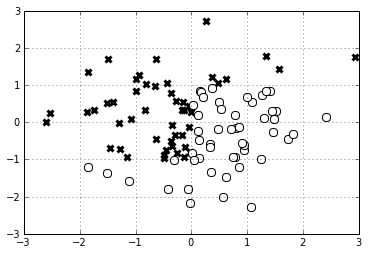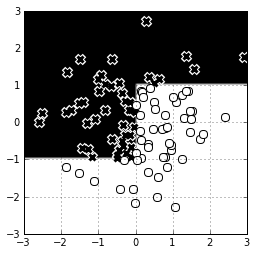In :
import numpy as np
import milk


Simulate some labeled data in a two-dimensional feature space.

In :
features = np.random.randn(100,2) # 2d array of features: 100 examples of 2 features each
labels = np.empty(100)
for i in range(100):
if features[i,0] < 0:
if features[i,1] < -1:
labels[i] = np.random.rand() < .001
else:
labels[i] = np.random.rand() < .999
else:
if features[i,1] < 1:
labels[i] = np.random.rand() < .001
else:
labels[i] = np.random.rand() < .999


What is the decision tree for this data?

Since the data is two-dimensional, we can take a look at it easily.

In :
plot(features[labels==True,0], features[labels==True,1], 'kx', mec='k', ms=6, mew=3)
plot(features[labels==False,0], features[labels==False,1], 'wo', mec='k', ms=8, mew=1)
grid()Fitting the model is easy:

In :
learner = milk.supervised.tree_learner()
model = learner.train(features, labels)


Using it is easy, too:

In :
model.apply([-1,1])

Out:
True

Visualizing the decision boundary is a bit of a pain...

In :
x_range = np.linspace(-3,3,100)
y_range = np.linspace(-3,3,100)

val = np.zeros((len(x_range), len(y_range)))

for i, x_i in enumerate(x_range):
for j, y_j in enumerate(y_range):
val[i,j] = model.apply([x_i,y_j])

imshow(val[::1,::-1].T, extent=[x_range,x_range[-1],y_range,y_range[-1]], cmap=cm.Greys)
plot(features[labels==True,0], features[labels==True,1], 'kx', mec='w', ms=7, mew=5)
plot(features[labels==True,0], features[labels==True,1], 'kx', mec='k', ms=5, mew=3)
plot(features[labels==False,0], features[labels==False,1], 'wo', mec='k', ms=8, mew=1)
grid()And can we have a picture of the decision tree itself? It is hidden in the model instance somewhere...

In :
model.tree

Out:
<milk.supervised.tree.Node at 0x1fc54850>
In :
model.tree.featid, model.tree.featval, model.tree.left, model.tree.right

Out:
(0,
-0.024916427900016365,
<milk.supervised.tree.Node at 0x1fc5ab50>,
<milk.supervised.tree.Node at 0x1fc54810>)
In :
def describe_tree(node, prefix=''):
print prefix + 'if x[%d] < %.2f:' % (node.featid, node.featval)
if isinstance(node.left, milk.supervised.tree.Node):
describe_tree(node.left, prefix+'    ')
else:
print prefix+'   ', node.left
print prefix + 'else:'
if isinstance(node.right, milk.supervised.tree.Node):
describe_tree(node.right, prefix+'    ')
else:
print prefix+'   ', node.right

In :
describe_tree(model.tree)

if x < -0.02:
if x < -0.97:
if x < -1.51:
Leaf(0.0,1.0)
else:
if x < -1.12:
Leaf(0.0,1.0)
else:
if x < -0.43:
Leaf(0.0,1.0)
else:
Leaf(0.0,3.0)
else:
if x < -0.99:
if x < -1.50:
if x < -2.53:
Leaf(1.0,1.0)
else:
if x < -1.87:
Leaf(1.0,1.0)
else:
if x < -1.85:
Leaf(1.0,1.0)
else:
Leaf(1.0,3.0)
else:
if x < -1.41:
Leaf(1.0,2.0)
else:
if x < -1.30:
Leaf(1.0,1.0)
else:
if x < -1.28:
Leaf(1.0,1.0)
else:
if x < -1.16:
Leaf(1.0,1.0)
else:
Leaf(1.0,3.0)
else:
if x < -0.94:
Leaf(1.0,1.0)
else:
if x < -0.63:
if x < -0.82:
Leaf(1.0,1.0)
else:
Leaf(1.0,3.0)
else:
if x < -0.37:
if x < -0.63:
Leaf(1.0,1.0)
else:
if x < -0.49:
Leaf(1.0,1.0)
else:
if x < -0.49:
Leaf(1.0,1.0)
else:
Leaf(1.0,3.0)
else:
if x < -0.25:
if x < -0.37:
Leaf(1.0,1.0)
else:
if x < -0.35:
Leaf(1.0,1.0)
else:
if x < -0.35:
Leaf(1.0,1.0)
else:
Leaf(1.0,3.0)
else:
if x < -0.13:
if x < -0.17:
Leaf(1.0,1.0)
else:
Leaf(1.0,3.0)
else:
if x < -0.13:
Leaf(1.0,1.0)
else:
if x < -0.12:
Leaf(1.0,1.0)
else:
if x < -0.05:
Leaf(1.0,1.0)
else:
Leaf(1.0,3.0)
else:
if x < 1.05:
if x < 0.00:
Leaf(0.5,2.0)
else:
if x < 0.79:
if x < 0.14:
if x < 0.02:
Leaf(0.0,1.0)
else:
if x < 0.04:
Leaf(0.0,1.0)
else:
if x < 0.11:
Leaf(0.0,1.0)
else:
Leaf(0.0,3.0)
else:
if x < 0.35:
if x < 0.15:
Leaf(0.0,1.0)
else:
if x < 0.18:
Leaf(0.0,1.0)
else:
if x < 0.21:
Leaf(0.0,1.0)
else:
Leaf(0.0,3.0)
else:
if x < 0.53:
if x < 0.38:
Leaf(0.0,1.0)
else:
Leaf(0.0,3.0)
else:
if x < 0.58:
Leaf(0.0,1.0)
else:
if x < 0.61:
Leaf(0.0,1.0)
else:
if x < 0.71:
Leaf(0.0,1.0)
else:
Leaf(0.0,3.0)
else:
if x < 0.95:
if x < 0.80:
Leaf(0.0,1.0)
else:
if x < 0.85:
Leaf(0.0,1.0)
else:
if x < 0.85:
Leaf(0.0,1.0)
else:
Leaf(0.0,3.0)
else:
if x < 1.31:
if x < 1.01:
Leaf(0.0,1.0)
else:
if x < 1.06:
Leaf(0.0,1.0)
else:
if x < 1.09:
Leaf(0.0,1.0)
else:
Leaf(0.0,3.0)
else:
if x < 1.46:
if x < 1.35:
Leaf(0.0,1.0)
else:
Leaf(0.0,3.0)
else:
if x < 1.48:
Leaf(0.0,1.0)
else:
if x < 1.51:
Leaf(0.0,1.0)
else:
if x < 1.73:
Leaf(0.0,1.0)
else:
Leaf(0.0,3.0)
else:
if x < 0.37:
Leaf(1.0,1.0)
else:
if x < 0.48:
Leaf(1.0,1.0)
else:
if x < 0.62:
Leaf(1.0,1.0)
else:
if x < 1.34:
Leaf(1.0,1.0)
else:
Leaf(1.0,3.0)


Not as simple as it seemed in the lecture, huh?